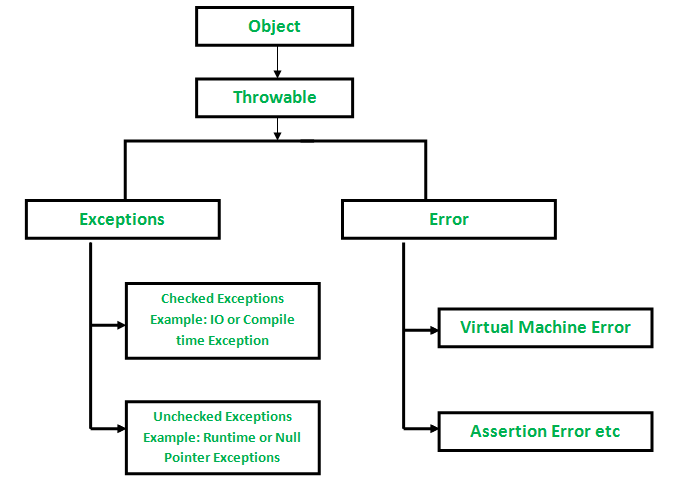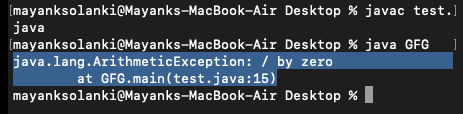Open in App
Not now

# Errors V/s Exceptions In Java

• Difficulty Level : Easy
• Last Updated : 24 Aug, 2022

In java, both Errors and Exceptions are the subclasses of java.lang.Throwable class. Error refers to an illegal operation performed by the user which results in the abnormal working of the program. Programming errors often remain undetected until the program is compiled or executed. Some of the errors inhibit the program from getting compiled or executed. Thus errors should be removed before compiling and executing. It is of three types:

• Compile-time
• Run-time
• Logical

Whereas exceptions in java refer to an unwanted or unexpected event, which occurs during the execution of a program i.e at run time, that disrupts the normal flow of the program’s instructions.Now let us discuss various types of errors in order to get a better understanding over arrays. As discussed in the header an error indicates serious problems that a reasonable application should not try to catch. Errors are conditions that cannot get recovered by any handling techniques. It surely causes termination of the program abnormally. Errors belong to unchecked type and mostly occur at runtime. Some of the examples of errors are Out of memory errors or System crash errors.

Example 1 Run-time Error

## Java

 `// Java Program to Illustrate Error``// Stack overflow error via infinite recursion` `// Class 1``class` `StackOverflow {` `    ``// method of this class``    ``public` `static` `void` `test(``int` `i)``    ``{``        ``// No correct as base condition leads to``        ``// non-stop recursion.``        ``if` `(i == ``0``)``            ``return``;``        ``else` `{``            ``test(i++);``        ``}``    ``}``}` `// Class 2``// Main class``public` `class` `GFG {` `    ``// Main driver method``    ``public` `static` `void` `main(String[] args)``    ``{``        ``// Testing for error by passing``        ``// custom integer as an argument``        ``StackOverflow.test(``5``);``    ``}``}`

Output:

Example 2

## Java

 `// Java Program to Illustrate Run-time Errors` `// Main class``class` `GFG {` `    ``// Main driver method``    ``public` `static` `void` `main(String args[])``    ``{` `        ``// Declaring and initializing numbers``        ``int` `a = ``2``, b = ``8``, c = ``6``;` `        ``if` `(a > b && a > c)``            ``System.out.println(a``                               ``+ ``" is the largest Number"``);``        ``else` `if` `(b > a && b > c)``            ``System.out.println(b``                               ``+ ``" is the smallest Number"``);` `        ``// The correct message should have been``        ``// System.out.println``        ``// (b+" is the largest Number"); to make logic``        ``else``            ``System.out.println(c``                               ``+ ``" is the largest Number"``);``    ``}``}`

Output

`8 is the smallest Number`

Now let us dwell onto Exceptions which indicates conditions that a reasonable application might want to catch. Exceptions are the conditions that occur at runtime and may cause the termination of the program. But they are recoverable using try, catch and throw keywords. Exceptions are divided into two categories:

Checked exceptions like IOException known to the compiler at compile time while unchecked exceptions like ArrayIndexOutOfBoundException known to the compiler at runtime. It is mostly caused by the program written by the programmer.

Example Exception

## Java

 `// Java program illustrating exception thrown``// by Arithmetic Exception class` `// Main class``class` `GFG {` `    ``// main driver method``    ``public` `static` `void` `main(String[] args)``    ``{``        ``int` `a = ``5``, b = ``0``;` `        ``// Try-catch block to check and handle exceptions``        ``try` `{` `            ``// Attempting to divide by zero``            ``int` `c = a / b;``        ``}``        ``catch` `(ArithmeticException e) {` `            ``// Displaying line number where exception occurred``            ``// using printStackTrace() method``            ``e.printStackTrace();``        ``}``    ``}``}`

Output:Finally now wrapping-off the article by plotting the differences out between them in a tabular format as provided below as follows:

My Personal Notes arrow_drop_up